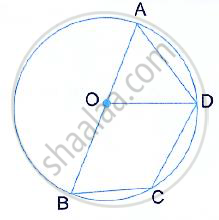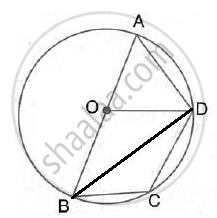Share

Ab is the Diameter of the Circle with Centre O. Od is Parallel to Bc and ∠Aod = 60°. Calculate the Numerical Values of : (I) ∠Abd, (Ii) ∠Dbc, (Iii) ∠Adc. - ICSE Class 10 - Mathematics

ConceptArc and Chord Properties - the Angle that an Arc of a Circle Subtends at the Center is Double that Which It Subtends at Any Point on the Remaining Part of the Circle

Question

AB is the diameter of the circle with centre O. OD is parallel to BC and ∠AOD = 60°. Calculate the numerical values of :
(i) ∠ABD, (ii) ∠DBC, (iii) ∠ADC.SolutionJoin BD.
(i)  ∠ABD = 1/2∠AOD = 1/2xx 60° = 30°
(Angle at the first is double the angle at the circumference subtended by the same chord)

(ii) ∠BDA = 90°
(Angle in a semicircle)

Also, ∠OAD is equilateral (∴ ∠OAD = 60° )

∴ ∠ODB = 90° - ∠ODA = 90° - 60° = 30°
Also, OD || BC
∴ ∠DBC = ∠ODB = 30° (Alternate angles)

(iii) ∠ABC = ∠ABD + ∠DBC = 30° + 30° = 60°
In cyclic quadrilateral ABCD,
∠ADC = 180° - ABC = 180° - 60° = 120°
(pair of opposite angles in a cyclic quadrilateral are supplementary)

Is there an error in this question or solution?

Video TutorialsVIEW ALL 

Solution Ab is the Diameter of the Circle with Centre O. Od is Parallel to Bc and ∠Aod = 60°. Calculate the Numerical Values of : (I) ∠Abd, (Ii) ∠Dbc, (Iii) ∠Adc. Concept: Arc and Chord Properties - the Angle that an Arc of a Circle Subtends at the Center is Double that Which It Subtends at Any Point on the Remaining Part of the Circle.
S